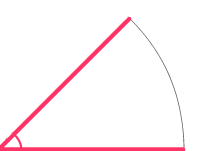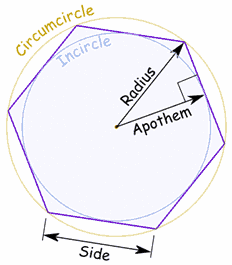A# geometry glossary

## acute

The word 'acute' comes from the Latin acus for 'needle' (which also forms the root for acid, acupuncture, and acumen). In mathematics, an acute angle is one that is less than 90°. An acute triangle is one in which all three interior angles are acute.## angle

See main article.

## apex

The apex is the angle of a polygon or polyhedron that is farthest from the side or plane designated the base of the figure. The apex of a cone or a pyramid is its vertex.

## apothem

The apothem, also known as a short radius, is the perpendicular distance from the center of a regular polygon to one of its sides. It is the same as the radius of a circle inscribed in the polygon.## area

See main article.

## central angle

The central angle is the angle subtended at the center of a circle by an arc or a chord; in other words, it is the angle between two radii.

## centroid

For a triangle, the centroid is the point of intersection of the medians (i.e., those lines drawn from each angle to the midpoint of the facing angle). For any other shape, the centroid is the point whose coordinates are the average of the coordinates of the shape's vertices. The centroid is the center of mass of a figure.

## circumcenter

The circumcenter is the center of a circle that passes through the vertices of a given polygon, usually a triangle. For a triangle, it is the same as the point of intersection of the perpendicular bisectors of the three sides. The point is equidistant from the triangle's vertices and hence the center of the circle that may be circumscribed about it (see circumscription).

## circumcircle

The circumcircle is the circle that passes through all three vertices of a given triangle. The circumcircle is said to circumscribe the triangle.

## circumference

The circumference is the curve which incloses a circle, ellipse, or other closed, plane figure. In figures bounded by straight lines, such as the triangle, square, or other type of polygon, the term perimeter is used to designate the sum of all the bounding lines taken together.

The length of the circumference depends partly on the nature of the curve; thus, that of the circle = 2πr = 2πd; and that of the ellipse = 2π[1 - (1/2)2e2/1 - (1 × 3/2 × 4)2e4/3 - (1 × 3 × 5/2 × 4 × 6)2e6/5 - ...], where a is the semi-major axis, and e the eccentricity.

The word comes the Latin circus ('circle') and ferre ('to carry'), and thus means literally "to carry around."

## circumscription

In plane geometry, circumscription is the construction of a circle such that all vertices of a particular polygon lie on its circumference, the polygon then being said to be inscribed within the circle. All regular polygons and all triangles may be circumscribed. In three dimensions circumscription implies the construction of a sphere such that on its surface lie all the vertices of a polyhedron.

## concave

See main article.

## congruent

In the case of geometric figures, congruent means having exactly the same shape and size, i.e., differing only in their positions in space. Two congruent figures will coincide exactly when superimposed. In contrast, two figures are said to be similar if the figures cannot be superimposed without being scaled or reflected.

## coplanar

Coplanar means lying in the same plane. It is possible to construct a plane through any set of three points, but this is true of sets of four points only in special cases. Any intersecting or parallel straight lines are coplanar.

## diagonal

a diagonal is a line that joins any two vertices of a polygon, if the vertices are not next to each other; or a line that joins two vertices of a polyhedron that are not on the same face.

## diameter

A diameter is a line joining any two points on the surface of a geometrical figure and passing through its center. The term is most often used in connection with the circle and sphere, all of whose infinitely many diameters have the same length.

## isosceles

A shape that is isosceles has two sides of the same length, as in the case of an isosceles triangle. An isosceles trapezoid in the United States is the equivalent of a trapezium in Britain. 'Isosceles' comes from the Greek iso (same or equal) and skelos (legs).

## perimeter

The perimeter is the distance around a two-dimensional shape, especially one bounded by straight lines, such as a triangle, square or polygon. In the case of a curved shape, such as a circle or ellipse, the term circumference is more commonly used.

## perpendicular

See main article.

## plane

See main article.

## straight

Something that is straight has no deviations. A straight line is usually simply called a line. A straight angle, or flat angle, is exactly 180°.

## vertex

A vertex is a point where two sides of a triangle (or other polygon), or two sides of an angle, intersect, or at which three or more sides of a pyramid or other polyhedron intersect; otherwise known as a corner. A cube, for example, has eight vertices. The point of a cone is also known as a vertex.